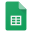# Statistical Process Control

## Central Limit Theorem

Empirical Proof of the CLT

The table on the google sheets contains 5 distributions of randomly assigned numbers that may be regenerated by refreshing the web Page (Reload).

Each row in the last column is the average/mean of the 5 randomly assigned columns.

The 5 Histograms of Distribution 1 to 5 are a visualization of the data distribution

The Histogram of Average is a visualization of the Average data.

1. Try refreshing the data and discuss whether the Distributions 1 to 5:

a. appear to have a Pattern or

b. it appears to be random

1. Discuss what you observe about the Histogram of Average?

a. Does there appear to be a pattern?

b.What does the pattern remind you of?

c. Do you as a group agree with the theory of the Central Limit Theorem found in https://en.wikipedia.org/wiki/Central_limit_theorem (the page is found below the Google Spreadsheet)Central Limit Theorem